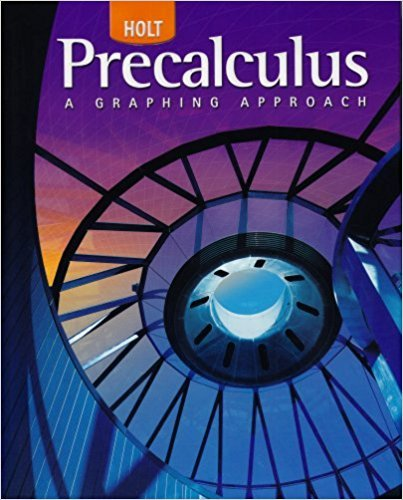×
×

# Solutions for Chapter 10.3: The Complex Plane and Polar Form for Complex Numbers## Full solutions for Precalculus | 1st Edition

ISBN: 9780030416477Solutions for Chapter 10.3: The Complex Plane and Polar Form for Complex Numbers

Solutions for Chapter 10.3
4 5 0 411 Reviews
28
4
##### ISBN: 9780030416477

Since 52 problems in chapter 10.3: The Complex Plane and Polar Form for Complex Numbers have been answered, more than 24427 students have viewed full step-by-step solutions from this chapter. This expansive textbook survival guide covers the following chapters and their solutions. Chapter 10.3: The Complex Plane and Polar Form for Complex Numbers includes 52 full step-by-step solutions. This textbook survival guide was created for the textbook: Precalculus, edition: 1. Precalculus was written by and is associated to the ISBN: 9780030416477.

Key Calculus Terms and definitions covered in this textbook
• Angle of depression

The acute angle formed by the line of sight (downward) and the horizontal

• Binomial coefficients

The numbers in Pascal’s triangle: nCr = anrb = n!r!1n - r2!

• Components of a vector

See Component form of a vector.

• Composition of functions

(f ? g) (x) = f (g(x))

• Degree of a polynomial (function)

The largest exponent on the variable in any of the terms of the polynomial (function)

• Dependent event

An event whose probability depends on another event already occurring

• Divergence

A sequence or series diverges if it does not converge

• Double-blind experiment

A blind experiment in which the researcher gathering data from the subjects is not told which subjects have received which treatment

• Intermediate Value Theorem

If ƒ is a polynomial function and a < b , then ƒ assumes every value between ƒ(a) and ƒ(b).

• Length of an arrow

See Magnitude of an arrow.

• Linear equation in x

An equation that can be written in the form ax + b = 0, where a and b are real numbers and a Z 0

• Midpoint (on a number line)

For the line segment with endpoints a and b, a + b2

• Parametric curve

The graph of parametric equations.

• Polar distance formula

The distance between the points with polar coordinates (r1, ?1 ) and (r2, ?2 ) = 2r 12 + r 22 - 2r1r2 cos 1?1 - ?22

• Right angle

A 90° angle.

• Second

Angle measure equal to 1/60 of a minute.

• Transpose of a matrix

The matrix AT obtained by interchanging the rows and columns of A.

• Transverse axis

The line segment whose endpoints are the vertices of a hyperbola.

• Window dimensions

The restrictions on x and y that specify a viewing window. See Viewing window.

• Zero of a function

A value in the domain of a function that makes the function value zero.

×